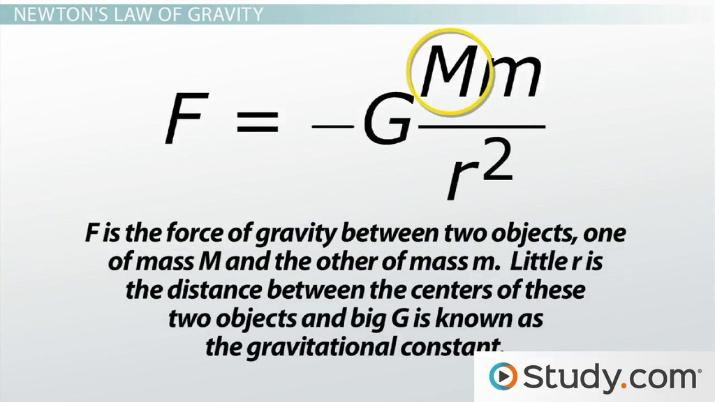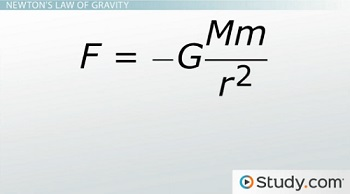# Sir Isaac Newton And Astronomy

Lesson Transcript
Instructor: Artem Cheprasov

Artem has a doctor of veterinary medicine degree.

Sir Isaac Newton was a mathematician and physicist who formulated three laws of motion for astronomy. Learn about the life of Sir Isaac Newton, Newton's laws of motion, Newton's law of gravity and how these laws affect orbital motion. Updated: 10/06/2021

## Sir Isaac Newton

You know you must be special if you have two birthdays! One such special individual was Sir Isaac Newton, an English mathematician and physicist. He was born on December 25, 1642. No, wait; it was January 4th, 1643. Actually, he was born on both dates. And it wasn't due to a spiritual re-awakening of some sort. It was because Protestant England used the Julian calendar, while the rest of Europe adopted the Gregorian calendar. Eh, whatever.

Newton was a bright child that attended college to study math and physics. But a plague swept through England and the colleges closed. So poor Newton had to meander back and study at home. Unfortunately, he didn't get much done as a result of this. He only managed to make discoveries in the field of optics, invent an entirely new mathematical field (that of calculus), and develop three laws of motion that changed the world - you know, normal college-student-on-a-break type stuff.An error occurred trying to load this video.

Try refreshing the page, or contact customer support.

Coming up next: Origins of the Julian & Gregorian Calendars

### You're on a roll. Keep up the good work!

Replay
Your next lesson will play in 10 seconds
• 0:03 Sir Isaac Newton
• 1:09 Newton's First Law of Motion
• 1:52 Newton's Second Law of Motion
• 2:29 Newton's Third Law of Motion
• 3:20 Newton's Law of Gravity
• 4:38 Newton and Orbital Motion
Save Save

Want to watch this again later?

Timeline
Autoplay
Autoplay
Speed Speed

## Newton's First Law of Motion

• Newton's first law of motion states that a body remains at rest or continues in uniform motion in a straight line unless acted upon by a force.

Thinking about this, Newton knew that something must pull our moon toward the Earth, meaning if no force altered the moon's motion, it would continue in a straight line and fly away into space. If we had no moon, that would really mess up the whole werewolf thing.

Anyways, Newton's thoughts point to the fact that the moon circles our Earth because the Earth attracts it through some force. Newton realized it was gravity that was this force.

## Newton's Second Law of Motion

• Newton's second law of motion states that the net force, F, on a body is equal to its acceleration, a, multiplied by its mass, m. In other words, F = ma.

The mass in the second law of motion is not the same thing as weight. Mass is a measurement of the amount of matter that makes up an object. An object's weight is actually the force that our Earth's gravity exerts on that object. Floating in space far away from Earth, you would have no weight despite having the same amount of matter as you have now on Earth.

## Newton's Third Law of Motion

• Newton's third law of motion says that when one body exerts a force on a second body, the second body exerts an equal and opposite force back on the first body.

But Newton also realized that the force between two bodies is dependent upon their masses and the distance between them.

As the square of the distance between two objects increases, the force of gravity decreases. Such a relationship is called an inverse square relation. For instance, if the distance from the Earth to the moon doubled, the gravitational force between the Earth and moon would decrease by 4, because 2 squared is equal to 4.

## Newton's Law of Gravity

Knowing what mass is and what an inverse square relation is, you can boil down Newton's law of gravity to the equation shown on the screen.In this equation, F is the force of gravity between two objects, one of mass M and the other of mass m. Little r is the distance between the centers of these two objects, and big G is known as the gravitational constant. The gravitational constant is a number that depends on the units we use for mass, force, and distance. The reason there's a minus sign in the equation is because the force of gravity is attractive, which means little r tends to decrease.

To unlock this lesson you must be a Study.com Member.

### Register to view this lesson

Are you a student or a teacher?

Back

### Resources created by teachers for teachers

Over 30,000 video lessons & teaching resources‐all in one place.Video lessonsQuizzes & WorksheetsClassroom IntegrationLesson Plans

I would definitely recommend Study.com to my colleagues. It’s like a teacher waved a magic wand and did the work for me. I feel like it’s a lifeline.

Jennifer B.
TeacherCreate an account to start this course today
Used by over 30 million students worldwide